# 通过仿真理解经典灰体辐射理论

2018年 11月 1日

### 经典热辐射理论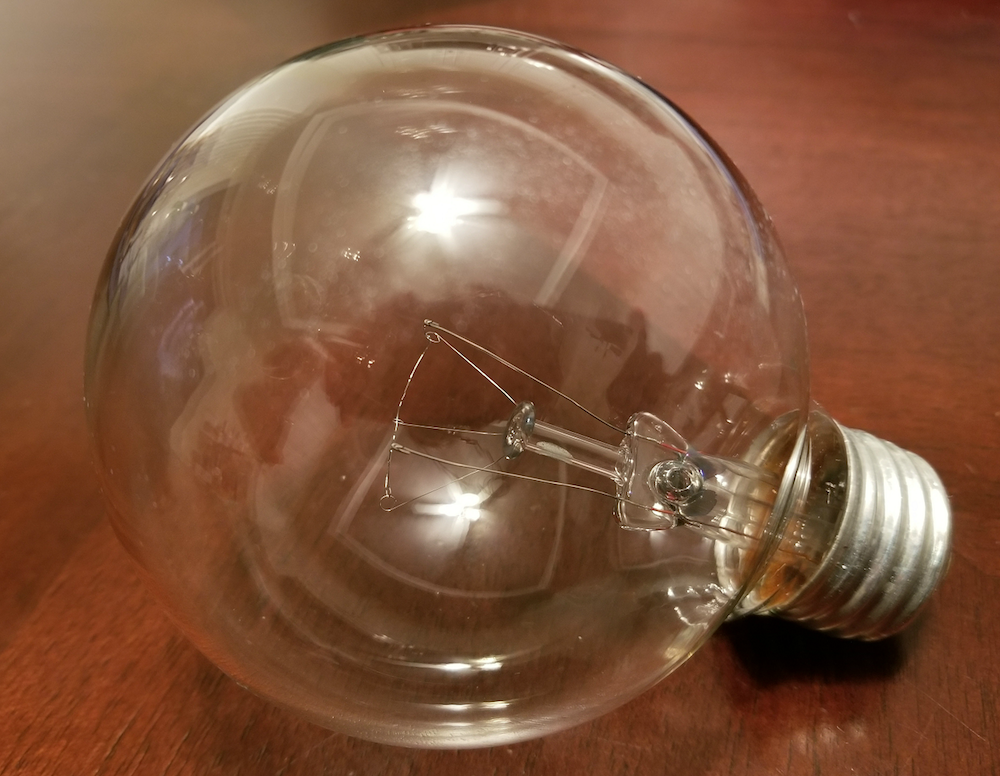I = n^2\sigma T^4 \hspace{1cm}(W/m^2),

• 材料反射率，\rho_0
• 材料吸收率，\alpha_0
• 材料发射率，\varepsilon_0
• 表观反射率，\rho
• 表观吸收率，\alpha
• 表观发射率，\varepsilon
• 腔体内部面积，S
• 开放面积，A
• 视角，\theta
• 角系数(归一化立体角)，F
• 角系数(面积比)，G

1= \rho+\alpha

\varepsilon = \alpha.

\varepsilon = 1-\rho.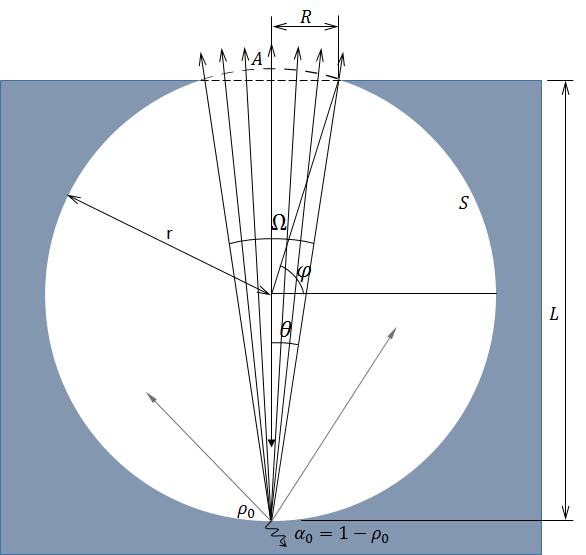\rho_0F,

\Omega = \int_0^\theta \int_0^{2\pi}\sin \theta ^\prime \cos \theta ^\prime d\theta ^\prime d\varphi ^\prime=\pi \sin^2\theta

F=\sin^2 \theta.

\rho^{(1)}=\rho_0 F,

\varepsilon^{(1)}= 1-\rho_0 F.

1-\rho_0F-\alpha_0= \rho_0(1-F)

\rho_0^2(1-F)G

\varepsilon^{(2)}=1-\rho_0F-\rho_0^2(1-F)G

\varepsilon^{(\infty)}=1-\rho_0F-\rho_0^2(1-F)G-\rho_0^3(1-F)G(1-G)-\rho_0^4(1-F)G(1-G)^2-\cdots =\frac{(1-\rho_0)( 1+\rho_0(G-F) ) }{1-\rho_0(1-G)}.

\varepsilon^{(\infty)}=\frac{1-\rho_0 } {1-\rho_0(1-G)}

F=\sin^2 \theta = \frac{R^2} {R^2+L^2}= \frac{1}{1+\left (\frac LR \right )^2}

A=\int_\varphi^{\pi/2} 2\pi r^2 \cos \varphi ^\prime \ d\varphi ^\prime = 2\pi r^2 (1-\sin \varphi)

A=2\pi r(2r-L)

G=\frac A S = \frac{2\pi r(2r-L)}{4\pi r^2} = \frac{1} {1+\left( \frac L R \right )^2},

### COMSOL Multiphysics® 仿真与理论比较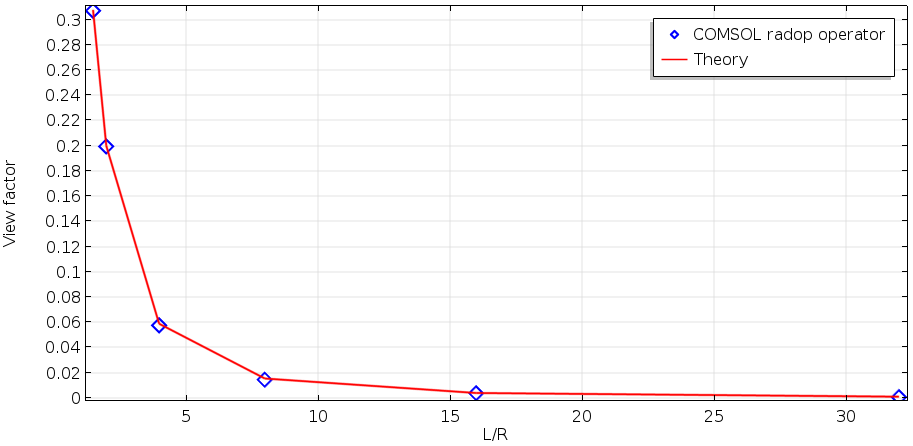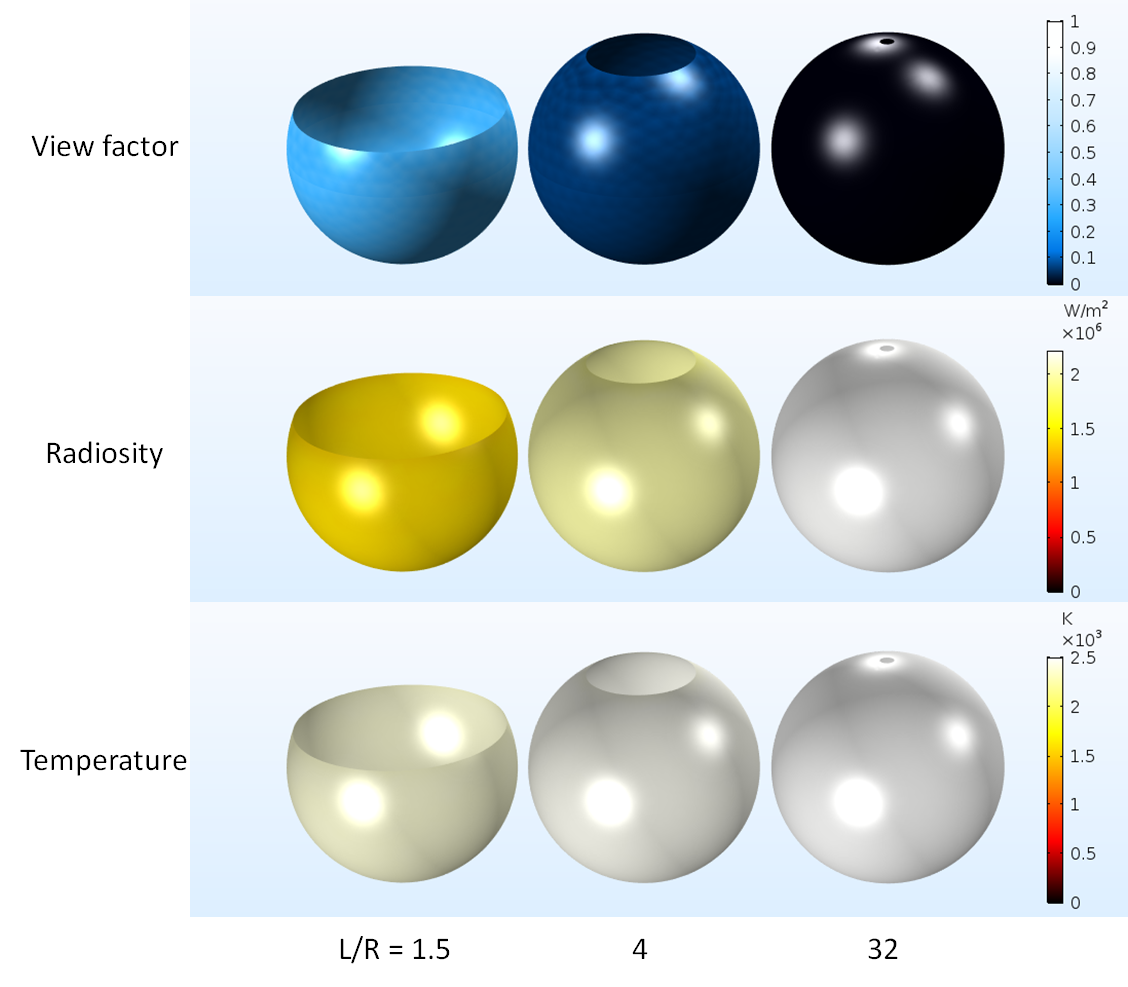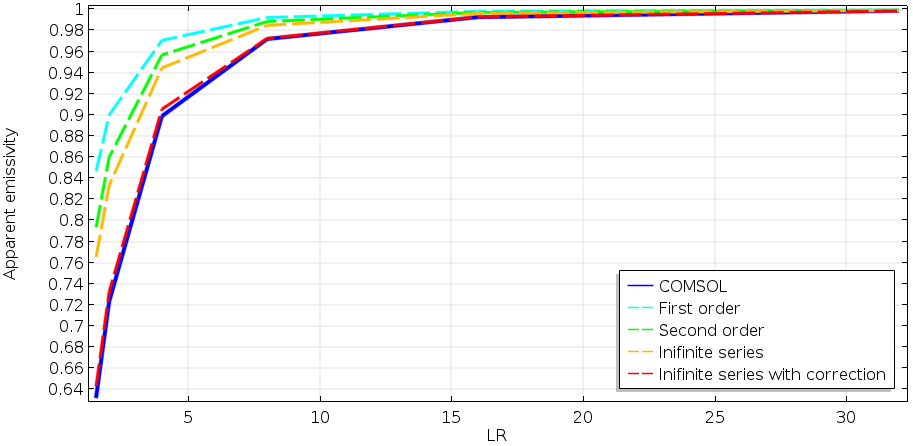Gouffé 论文的最后一部分提到了导致这种差异的原因。正如一些读者可能已经注意到，上图中每个几何形状的表面温度都不同，尽管外部的边界温度相同，为 2500 K。这称为辐射冷却。我们还没有包括这种效应，它发生在任何具有有限热导率的真实材料上。辐射冷却效应会改变外壳温度的内表面温度，具体取决于开口面积。因此，需要对温度进行补偿，以计算所有开口区域在相同温度下的发射率。

### 使用 COMSOL® 软件设计高效白炽灯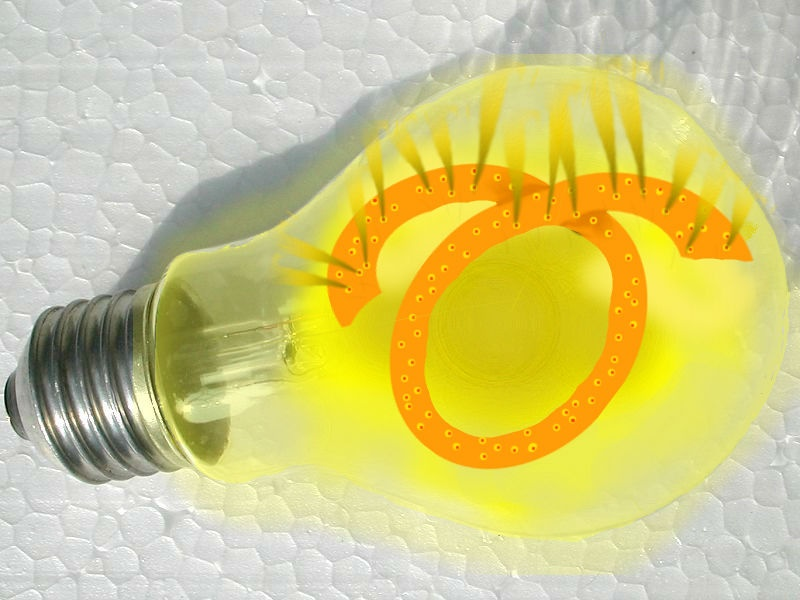### 参考文献

1. A. Gouffé, “Corrections d’ouverture des corps-noirs artificiels compte tenu des diffusions multiples internes (Corrections of emissivity for the artificial black-body considering multiple internal diffusions)” (in French), Revue d’Optique, t. 24, no. 1–3, 1945.
2. C.J. Smithells, Tungsten, Chapman & Hall, Ltd., 1926.
3. J.F. Waymouth, Proceedings of LS-5, 1989.
4. J.F. Waymouth, J. Illum. Engng. Inst. Jpn., 74, 12, pp. 800–805, 1990.
5. J.R. Howell, “A Catalog of Radiation Heat Transfer Configuration Factors“, University of Texas at Austin.
6. W.Z. Black, “Radiative heat transfer characteristics of specially prepared V-groove cavities”, PhD Thesis to Purdue University, 1968.
7. Precision Measurement and Calibration, Radiometry and Photometry (Vol. 7), U.S. Department of Commerce, National Bureau of Standards.
8. M. Sugimoto, T. Fujioka, T. Inoue, H. Fukushima, Y. Mizuyama, S. Ukegawa, T. Matsushima, and M. Toho, “The Infrared Suppression in the Incandescent Light from a Surface with Submicron Holes”, Journal of Light & Visual Environment, Vol. 18, No. 2 (1994).
9. T. Kondo, S. Hasegawa, T. Yanagishita, N. Kimura, T. Toyonaga, and H. Masuda, “Control of thermal radiation in metal hole array structures formed by anisotropic anodic etching of Al”, Optics Express Vol. 26 No. 21 (2018).